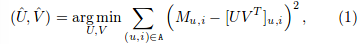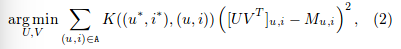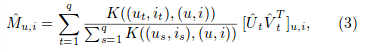### Local low-rank matrix approximationLocal的Local组合成最终的model##### 留言

• 文献基本信息
 文献题目 去谷歌学术搜索 Local low-rank matrix approximation 文献作者 Joonseok Lee; Seungyeon Kim; Guy Lebanon 文献发表年限 2013 文献关键字 摘要描述 Matrix approximation is a common tool in recommendation systems, text mining, and computer vision. A prevalent assumption in constructing matrix approximations is that the partially observed matrix is of low-rank. We propose a new matrix approximation model where we assume instead that the matrix is locally of low-rank, leading to a representation of the observed matrix as a weighted sum of low-rank matrices. We analyze the accuracy of the proposed local low-rank modeling. Our experiments show improvements in prediction accuracy over classical approaches for recommendation tasks.
• 博主信息Nengjun 内部成员